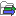# Convert Abundance Table to Experiment

Most statistical tools take abundance tables as input, but the ones listed below require an experiment table:

• Empirical Analysis of DGE (legacy) (). Please use now the Differential Abundance Analysis.

• Hierarchical Clustering of Samples () with which the data needed to be normalized before hand. Please use now the Create Heat Map for Abundance Table that does not require any normalization prior to its use.

The Convert Abundance Table to Experiment allows you to transform an abundance table into an experiment table, allowing users to use the thus-generated table to perform the statistical tests listed above.

To use the tool, go to:

Metagenomics | Abundance Analysis () | Convert Abundance Table to Experiment ()

Choose an abundance table as input, and define which metadata group is to be considered as factor.

The tool outputs a table labeled (experiment). The first column is the name of the group used as factor in the analysis (its taxonomy and an ID number). For each feature (e.g. an OTU, an organism, a Pfam family or a GO term) there will be the following data:

• Range: The difference between the highest and the lowest expression value for the feature over all the samples.
• IQR: The inter-quantile range of the values for a feature across the samples, that is, the difference between the 75%-ile value and the 25%-ile value.
• Difference: The difference for a two-group experiment between the mean of the expression values across the samples assigned to group 2 and the mean of the expression values across the samples assigned to group 1. Thus, if the mean expression level in group 2 is higher than that of group 1 the 'Difference' is positive, and if it is lower the 'Difference' is negative. For experiments with more than two groups the 'Difference' contains the difference between the maximum and minimum of the mean expression values of the groups, multiplied by -1 if the group with the maximum mean expression value occurs before the group with the minimum mean expression value (with the ordering: group 1, group 2, ...).
• Fold change: For a two-group experiment the 'Fold Change' tells you how many times bigger the mean expression value in group 2 is relative to that of group 1. If the mean expression value in group 2 is bigger than that in group 1 this value is the mean expression value in group 2 divided by that in group 1. If the mean expression value in group 2 is smaller than that in group 1 the fold change is the mean expression value in group 1 divided by that in group 2 with a negative sign. Thus, if the mean expression levels in group 1 and group 2 are 10 and 50 respectively, the fold change is 5, and if the and if the mean expression levels in group 1 and group 2 are 50 and 10 respectively, the fold change is -5. For experiments with more than two groups, the 'Fold Change' column contains the ratio of the maximum of the mean expression values of the groups to the minimum of the mean expression values of the groups, multiplied by -1 if the group with the maximum mean expression value occurs before the group with the minimum mean expression value (with the ordering: group 1, group 2, ...).
• Taxonomy: The taxonomy of the feature, if specified. For OTUs, this is specified by the reference database when a database entry was used as Reference.
• Expression values and Means for each site: The expression values represents the number of reads belonging to the feature in a specific sample, and Means is the mean of these expression values.

You can create a sub-experiment by selecting only some of the features from your experiment table (the most abundant ones for example).